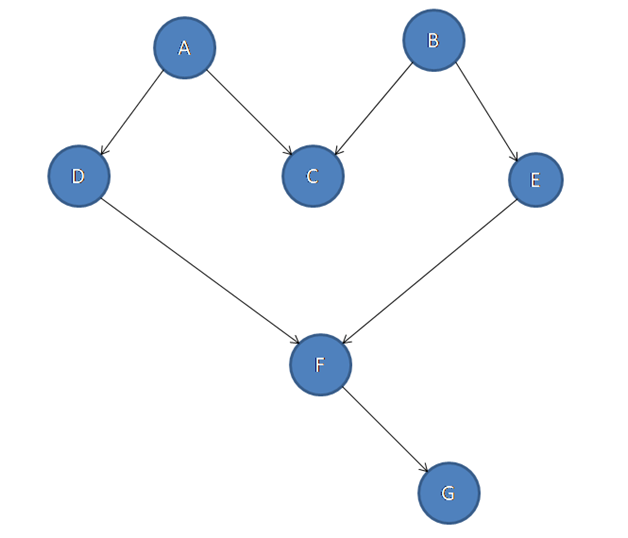# Topological sort implementation using C++ program

Topological sort implementation: Here, we are going to implement Topological sort using C ++ program.
Submitted by Souvik Saha, on May 08, 2019

Problem statement:

Given a graph of n vertices, you have to topologically sort that graph.

Example:

Input:

If there is graph be like the below:Output: A → B → C → D → E → F → G

## Topological sort

It is a technique where each node comes after it's parent node.

Here, node A and node B are two nodes which have no parents. So A comes first then D node comes but to print F we need D and E both the nodes so it is not possible. Then comes node B and node C also available and then E, F and G come.

Algorithm:

To solve this problem we follow this process:

1. We take two stacks and a set. We use the set to mark the nodes.
2. We start with any of the vertexes and push it into the stack and mark it (insert into the set).
3. We take the top element and check for its unvisited adjacent vertices if it exists then push it into the stack and if not then all the nodes are visited therefore we pop that node from the stack and place it into the other stack.
4. We repeat step 3 until we visited all the nodes.

C++ implementation:

```#include <bits/stdc++.h>
using namespace std;

void addedge(list<int>* ls, int x, int y)
{
ls[x].push_back(y);
}

string topological_sort(list<int>* ls, int k)
{
char arr[k];
stack<int> s;
set<int> st;
int ind = k - 1;
for (int i = k - 1; i >= 0; i--) {
if (st.find(i) == st.end()) {
s.push(i);
st.insert(i);
//check all the non visited nodes
while (!s.empty()) {
int p = s.top();
list<int>::iterator it;
int temp = 0;
//check its adjacent non visited nodes
for (it = ls[p].begin(); it != ls[p].end(); it++) {
if (st.find(*it) == st.end()) {
st.insert(*it);
s.push(*it);
temp = 1;
}
}
//if all adjaceny nodes are visited then pop that element from stack
if (temp == 0) {
char ch = (char)(p + 'A');
arr[ind] = ch;
ind--;
s.pop();
}
}
}
}
return arr;
}
int main()
{
int k = 7;
list<int> ls[k];
cout << topological_sort(ls, 7) << endl;
return 0;
}
```

Output

```ABCDEFG
```